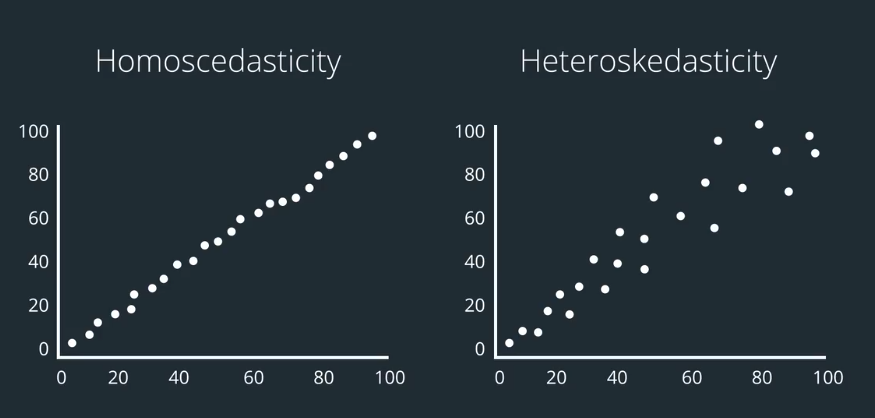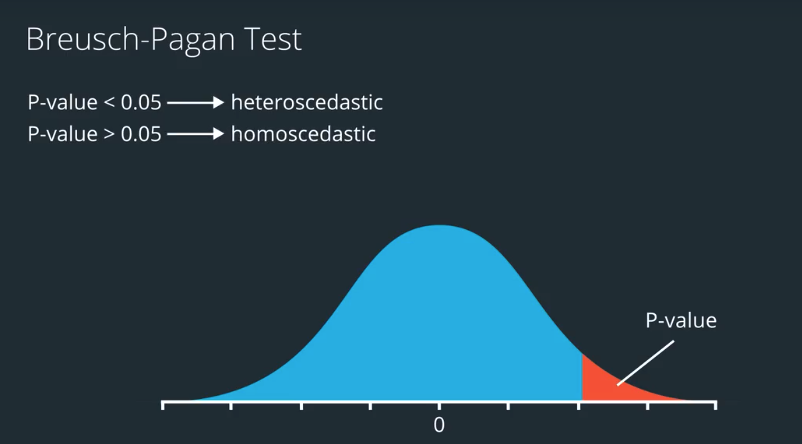# AI For Trading: Heteroskedasticity (18)

### Heteroskedasticity

One of the assumptions of linear regression is that its input data are homoscedastic(同方差的). A visual way to check if the our data is homoscedastic is a scatter plot (like the one we saw in the video).

If our data is heteroscedastic(异方差性), a linear regression estimate of the coefficients may be less accurate (further from the actual value), and we may get a smaller p-value than should be expected, which means we may assume (incorrectly) that we have an accurate estimate of the regression coefficient, and assume that it’s statistically significant when it’s not.### Breusch-Pagan Test

Note, we’ll cover the Breusch-Pagan test for heteroscedasticity in more detail after we learn about regression.